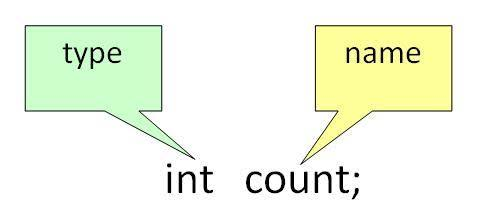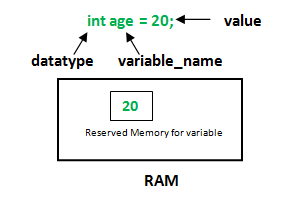# Variables in Java

A variable is a name given to a memory location. It is the basic unit of storage in a program.

• The value stored in a variable can be changed during program execution.
• A variable is only a name given to a memory location, all the operations done on the variable effects that memory location.
• In Java, all the variables must be declared before use.

How to declare variables?

We can declare variables in java as follows:type: Type of data that can be stored in this variable.
name: Name given to the variable.
In this way, a name can only be given to a memory location. It can be assigned values in two ways:

• Variable Initialization
• Assigning value by taking input

How to initialize variables?datatype: Type of data that can be stored in this variable.
variable_name: Name given to the variable.
value: It is the initial value stored in the variable.

Examples:

```float simpleInterest; //Declaring float variable
int time = 10, speed = 20; //Declaring and Initializing integer variable
char var = 'h'; // Declaring and Initializing character variable
```

Types of variables

There are three types of variables in Java:

• Local Variables
• Instance Variables
• Static Variables

Let us now learn about each one of these variables in detail.

1. Local Variables: A variable defined within a block or method or constructor is called local variable.

• These variable are created when the block in entered or the function is called and destroyed after exiting from the block or when the call returns from the function.
• The scope of these variables exists only within the block in which the variable is declared. i.e. we can access these variable only within that block.
• Initilisation of Local Variable is Mandatory.

Sample Program 1:

 `public` `class` `StudentDetails { ` `    ``public` `void` `StudentAge() ` `    ``{ ` `        ``// local variable age ` `        ``int` `age = ``0``; ` `        ``age = age + ``5``; ` `        ``System.out.println(``"Student age is : "` `+ age); ` `    ``} ` ` `  `    ``public` `static` `void` `main(String args[]) ` `    ``{ ` `        ``StudentDetails obj = ``new` `StudentDetails(); ` `        ``obj.StudentAge(); ` `    ``} ` `} `

Output:

```Student age is : 5
```

In the above program, the variable age is a local variable to the function StudentAge(). If we use the variable age outside StudentAge() function, the compiler will produce an error as shown in below program.

Sample Program 2:

 `public` `class` `StudentDetails { ` `    ``public` `void` `StudentAge() ` `    ``{ ``// local variable age ` `        ``int` `age = ``0``; ` `        ``age = age + ``5``; ` `    ``} ` ` `  `    ``public` `static` `void` `main(String args[]) ` `    ``{ ` `        ``// using local variable age outside it's scope ` `        ``System.out.println(``"Student age is : "` `+ age); ` `    ``} ` `} `

Output:

```Compilation Error in java code :-
prog.java:12: error: cannot find symbol
System.out.println("Student age is : " + age);
^
symbol:   variable age
location: class StudentDetails
1 error
```
2. Instance Variables: Instance variables are non-static variables and are declared in a class outside any method, constructor or block.

• As instance variables are declared in a class, these variables are created when an object of the class is created and destroyed when the object is destroyed.
• Unlike local variables, we may use access specifiers for instance variables. If we do not specify any access specifier then the default access specifier will be used.
• Initilisation of Instance Variable is not Mandatory. Its default value is 0
• Instance Variable can be accessed only by creating objects.

Sample Program:

 `import` `java.io.*; ` `class` `Marks { ` `    ``// These variables are instance variables. ` `    ``// These variables are in a class ` `    ``// and are not inside any function ` `    ``int` `engMarks; ` `    ``int` `mathsMarks; ` `    ``int` `phyMarks; ` `} ` ` `  `class` `MarksDemo { ` `    ``public` `static` `void` `main(String args[]) ` `    ``{ ` `        ``// first object ` `        ``Marks obj1 = ``new` `Marks(); ` `        ``obj1.engMarks = ``50``; ` `        ``obj1.mathsMarks = ``80``; ` `        ``obj1.phyMarks = ``90``; ` ` `  `        ``// second object ` `        ``Marks obj2 = ``new` `Marks(); ` `        ``obj2.engMarks = ``80``; ` `        ``obj2.mathsMarks = ``60``; ` `        ``obj2.phyMarks = ``85``; ` ` `  `        ``// displaying marks for first object ` `        ``System.out.println(``"Marks for first object:"``); ` `        ``System.out.println(obj1.engMarks); ` `        ``System.out.println(obj1.mathsMarks); ` `        ``System.out.println(obj1.phyMarks); ` ` `  `        ``// displaying marks for second object ` `        ``System.out.println(``"Marks for second object:"``); ` `        ``System.out.println(obj2.engMarks); ` `        ``System.out.println(obj2.mathsMarks); ` `        ``System.out.println(obj2.phyMarks); ` `    ``} ` `} `

Output:

```Marks for first object:
50
80
90
Marks for second object:
80
60
85
```

As you can see in the above program the variables, engMarks , mathsMarks , phyMarksare instance variables. In case we have multiple objects as in the above program, each object will have its own copies of instance variables. It is clear from the above output that each object will have its own copy of instance variable.

3. Static Variables: Static variables are also known as Class variables.

• These variables are declared similarly as instance variables, the difference is that static variables are declared using the static keyword within a class outside any method constructor or block.
• Unlike instance variables, we can only have one copy of a static variable per class irrespective of how many objects we create.
• Static variables are created at the start of program execution and destroyed automatically when execution ends.
• Initilisation of Static Variable is not Mandatory. Its default value is 0
• If we access the static variable like Instance variable (through an object), the compiler will show the warning message and it won’t halt the program. The compiler will replace the object name to class name automatically.
• If we access the static variable without the class name, Compiler will automatically append the class name.

To access static variables, we need not create an object of that class, we can simply access the variable as

`class_name.variable_name;`

Sample Program:

 `import` `java.io.*; ` `class` `Emp { ` ` `  `    ``// static variable salary ` `    ``public` `static` `double` `salary; ` `    ``public` `static` `String name = ``"Harsh"``; ` `} ` ` `  `public` `class` `EmpDemo { ` `    ``public` `static` `void` `main(String args[]) ` `    ``{ ` ` `  `        ``// accessing static variable without object ` `        ``Emp.salary = ``1000``; ` `        ``System.out.println(Emp.name + ``"'s average salary:"` `                           ``+ Emp.salary); ` `    ``} ` `} `

Output:

```Harsh's average salary:1000.0
```

Instance variable Vs Static variable

• Each object will have its own copy of instance variable whereas We can only have one copy of a static variable per class irrespective of how many objects we create.
• Changes made in an instance variable using one object will not be reflected in other objects as each object has its own copy of instance variable. In case of static, changes will be reflected in other objects as static variables are common to all object of a class.
• We can access instance variables through object references and Static Variables can be accessed directly using class name.
• Syntax for static and instance variables:
```class Example
{
static int a; //static variable
int b;        //instance variable
}
```

Similar Articles:

This article is contributed by Harsh Agarwal. If you like GeeksforGeeks and would like to contribute, you can also write an article using contribute.geeksforgeeks.org or mail your article to contribute@geeksforgeeks.org. See your article appearing on the GeeksforGeeks main page and help other Geeks.

Attention reader! Don’t stop learning now. Get hold of all the important Java and Collections concepts with the Fundamentals of Java and Java Collections Course at a student-friendly price and become industry ready.

My Personal Notes arrow_drop_up

Article Tags :
Practice Tags :

51

Please write to us at contribute@geeksforgeeks.org to report any issue with the above content.